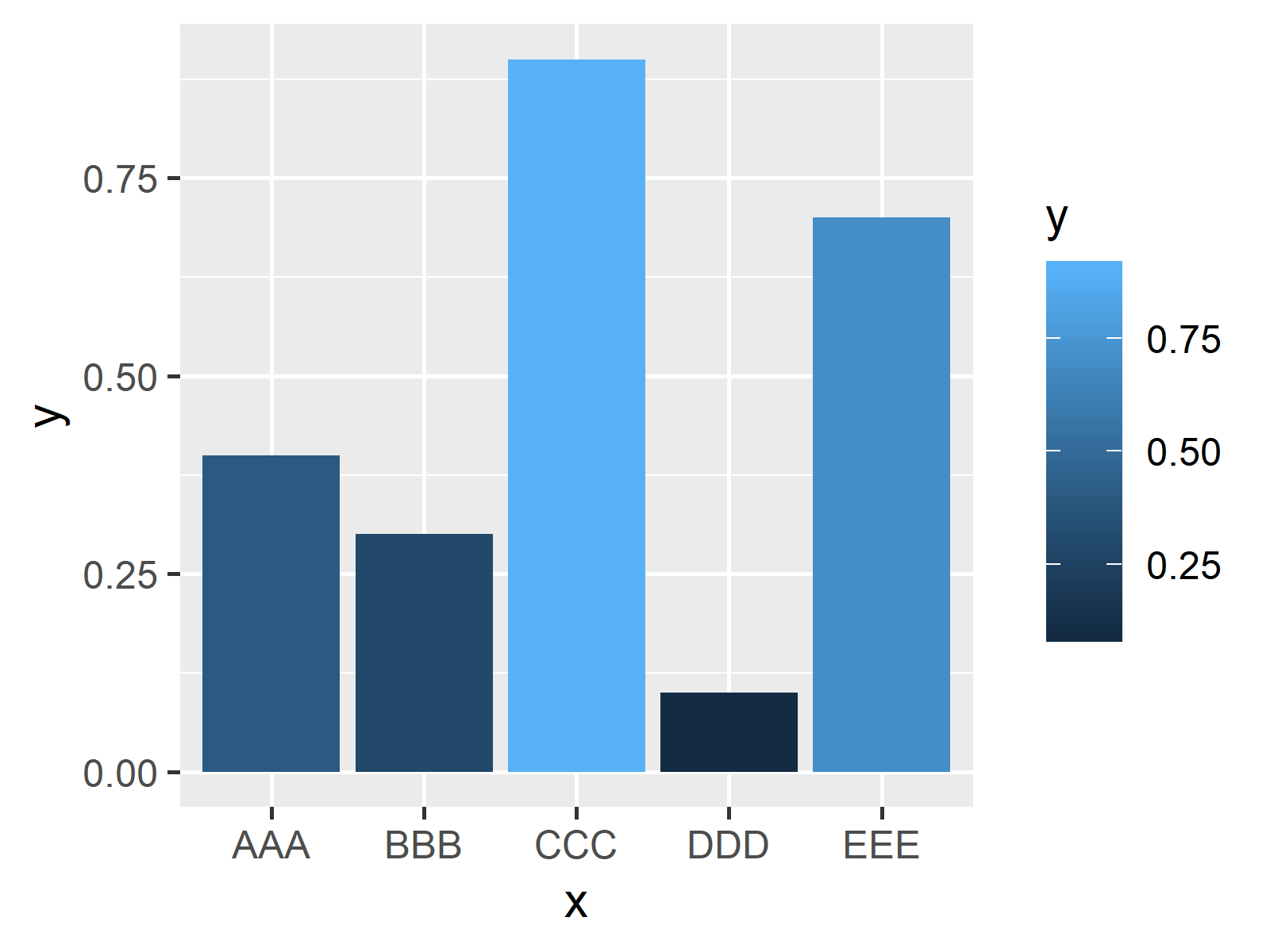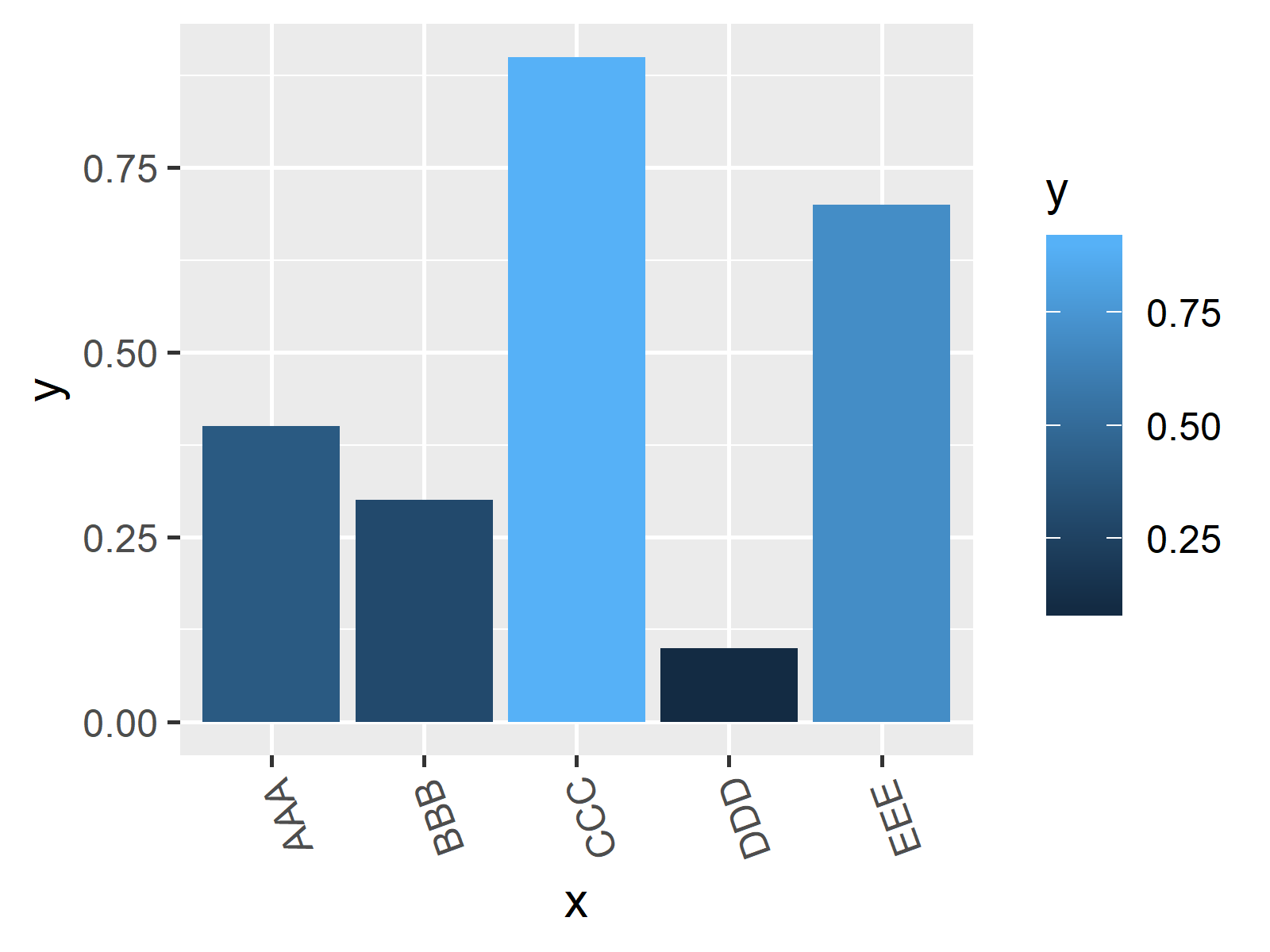# Rotate ggplot2 Axis Labels in R (2 Examples)

This article explains how to rotate the axis labels of a ggplot in the R programming language.

The article contains the following topics:

So now the part you have been waiting for – the examples!

## Creation of Example Data & Basic Plot

In the examples of this tutorial, I’m going to use the following data:

```data <- data.frame(x = c("AAA", "BBB", "CCC", "DDD", "EEE"),  # Create example data
y = c(0.4, 0.3, 0.9, 0.1, 0.7))```

Let’s see how this data looks like in a ggplot2 plot with default specifications. First, we need to install and load the ggplot2 R package

```install.packages("ggplot2")                                   # Install ggplot2 package

…and then we can plot our example data in a barchart:

```ggplot(data, aes(x, y, fill = y)) +                           # ggplot2 with default settings
geom_bar(stat = "identity")```Figure 1: Basic ggplot2 Barchart with Default Specifications.

Figure 1 illustrates how our basic barchart with default specifications looks like. Note that we could use any other type of ggplot2 graphic or diagram (e.g. histogram, scatterplot, boxplot etc.) for the following examples.

## Example 1: Rotate ggplot with 90 Degree Angle

If we want to set our axis labels to a vertical angle, we can use the theme & element_text functions of the ggplot2 package. We simply have to add the last line of the following R code to our example plot:

```ggplot(data, aes(x, y, fill = y)) +
geom_bar(stat = "identity") +
theme(axis.text.x = element_text(angle = 90))               # Rotate axis labels```Figure 2: Barchart with 90 Degree Angle.

As you can see based on Figure 2, the x-axis text was changed to a vertical angle. Note that we could apply the same approach to the y-axis by using axis.text.y instead of axis.text.x within the theme function.

## Example 2: Rotate ggplot with Other Angles

In the previous example, we rotated our plot axis labels with a 90 degree angle. However, we could specify basically any angle we want by changing the value that we assign to the angle argument within the element_text function.

For instance, we could use a 110 degree angle:

```ggplot(data, aes(x, y, fill = y)) +
geom_bar(stat = "identity") +
theme(axis.text.x = element_text(angle = 110))               # Rotate axis labels unevenly```Figure 3: Barchart with Highly Rotated X-Axis.

## Video & Further Resources

If you want to learn more on rotating axis labels in ggplots, you could have a look at the following YouTube video of the Statistics Globe YouTube channel. In the video, I’m showing the examples of this page in a live session:

Please accept YouTube cookies to play this video. By accepting you will be accessing content from YouTube, a service provided by an external third party.If you accept this notice, your choice will be saved and the page will refresh.

Furthermore, you might have a look at the other R tutorials of my website. I have published several related ggplot2 tutorials already:

In this article, I showed you how to change the angle of axis labels in the R programming language. If you have any comments or questions, let me know in the comments section below.

Subscribe to the Statistics Globe Newsletter